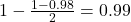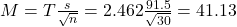## A random sample of 30 households was selected as part of a study on electricity usage, and the number of kilowatt-hours (kWh) was recorded f

Question

A random sample of 30 households was selected as part of a study on electricity usage, and the number of kilowatt-hours (kWh) was recorded for each household in the sample for the March quarter of 2006. The average usage was found to be 375kWh. It is believed that the standard deviation from the population has changed and thus is unknown. However, from the small data set in 2006, we know the sample standard deviation is 91.5kWh. Assuming that the usage is normally distributed, provide an expression for calculating a 98% confidence interval for the mean usage in the March quarter of 2006.

in progress 0
2 months 2021-07-31T00:55:22+00:00 1 Answers 2 views 0

The 98% confidence interval for the mean usage in the March quarter of 2006, in kWh, was (333.87, 416.13).

Step-by-step explanation:

We have the standard deviation for the sample, which means that the t-distribution is used to solve this question.

The first step to solve this problem is finding how many degrees of freedom, we have. This is the sample size subtracted by 1. So

df = 30 – 1 = 29

98% confidence interval

Now, we have to find a value of T, which is found looking at the t table, with 29 degrees of freedom(y-axis) and a confidence level of. So we have T = 2.462

The margin of error is:In which s is the standard deviation of the sample and n is the size of the sample.

The lower end of the interval is the sample mean subtracted by M. So it is 375 – 41.13 = 333.87 kWh

The upper end of the interval is the sample mean added to M. So it is 375 + 41.13 = 416.13 kWh

The 98% confidence interval for the mean usage in the March quarter of 2006, in kWh, was (333.87, 416.13).